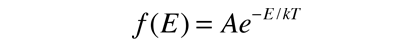# Average Molecular Kinetic Energy

The molecules of matter at ordinary temperatures can be considered to be in ceaseless, random motion at high speeds. The average translational kinetic energy for these molecules can be deduced from the Boltzmann distribution. When the Boltzmann distributionis applied to the motion of a molecule in one dimension, it becomesShow

This distribution function can be used to calculate the average value of the square of the velocity.This integral can be put in the standard form:This gives:The average kinetic energy for one dimension is thenand for three dimensions of such motion the average kinetic energy is:We can with confidence just multiply the one-dimensional result by three since the different components of velocity are independent of each other. This assignment of kT/2 of energy to each degree of freedom of the molecule's motion is called equipartition of energy. This microscopic kinetic energy is often called "thermal energy" and this expression is useful in defining the kinetic temperature.

Note that the average kinetic energy for molecules is not the same as the average energy for purely random energies under the Boltzmann distribution, which is Eavg=kT. If you consider all velocities to be equally probable, then all velocities within a range v to v+Δv can be considered to form a spherical shell in "velocity space". For a larger value of v, the shell is bigger and there are more possibilities. This tips the average kinetic energy to a higher value, which turns out to be 3kT/2 instead of kT. This kind of consideration arises in the development of the Maxwell speed distribution for molecules.

 Applications of the Boltzmann distribution
Index

Kinetic theory concepts

 HyperPhysics***** Thermodynamics R Nave
Go Back

# Average Energy Integral: Boltzmann Distribution

The average energy integral for the distribution of energy among a collection of particles according to the Boltzmann distribution is:This integral may be evaluated using integration by parts.This technique is particularly appropriate for removing a linear term multiplying an exponential.Index

Kinetic theory concepts

 HyperPhysics***** Thermodynamics R Nave
Go Back